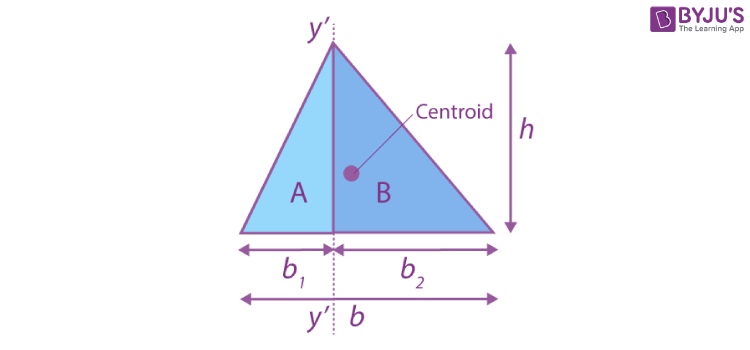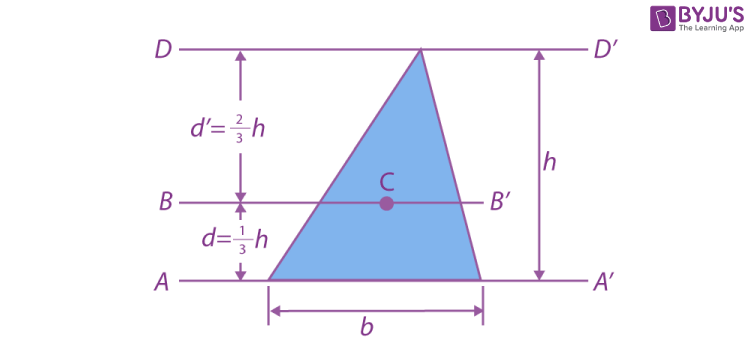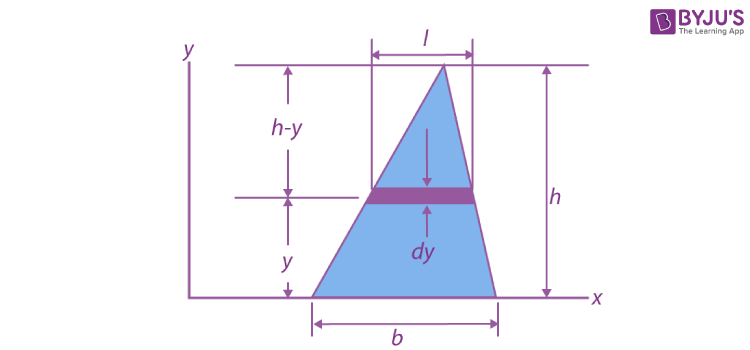Checkout JEE MAINS 2022 Question Paper Analysis : Checkout JEE MAINS 2022 Question Paper Analysis :

# Moment Of Inertia Of A Triangle

Moment of inertia of a triangle can be expressed in various ways. There are usually three moments that can be considered. They are;

• Axis passing through the centroid.
• Axis passing through the base.
• The axis perpendicular to its base.

We will look at each expression below.

1. Axis passing through the centroid

The moment of inertia of a triangle having its axis passing through the centroid and parallel to its base is expressed as;

 I = bh3 / 36

Here, b = base width and h = height

2. Axis passing through the base

If we take the axis that passes through the base, the moment of inertia of a triangle is given as;

 I = bh3 / 12

We can further use the parallel axis theorem to prove the expression where the triangle centroid is located or found at a distance equal to h/3 from the base.

3. The axis perpendicular to its base

When we want to determine the moment of inertia of a triangle when its axis is perpendicular to its base we have to first consider that axis y’-y’ is used in dividing the whole triangle into two right triangles respectively A and B. However, these triangles will have a common base equal to h, and heights b1 and b2. The moment of inertia for both will be:

Iy’ = hb13 / 12 + hb23 / 12

If we consider b2 = b – b1 where the parallel axis y-y through the centroid is at a distance ⅔ ( b / 2 – b1) from y’-y’ then we can easily find or calculate the moment of inertia ly. We can use the parallel axis theorem to do so. In any case, after algebraic substitutions, we get the expression as:

 Iy’ = (hb / 36) (b2 – b1 b + b12)## Calculating Moment Of Inertia Of A Triangle

We will take the case where we have to determine the moment of inertia about the centroid y. We will consider the moment of inertia y about the x-axis.We will use the parallel axis theorem and we will take the centroid as a reference in this case.

Here,

= (1/12) bh3 – 1/2 bh (1/3) h2

= bh3 / 36

Next, we will find the moment of inertia when the axis passes through its base.We will consider the differential strip which is parallel to the x-axis for da. It is given as;

dlx = y2 dA

dA = l dy

If we take similar triangles we will have,

(l / b) = (h – y) / h

l = b (h – y )/ h

dA = b [(h – y) / h]dy

Now we will integrate dlx from y = 0 to y = h.

Ix = ∫ y2 dA

Ix = Oh y2 b (h – y)/ h dy

Ix = b/h Oh (hy2 – y3) dy

Ix = (b/h)[h (y3/ 3)- (y4 / 4) ]0h

Therefore, Ix = bh3 / 12

### Parallel Axis Theorem